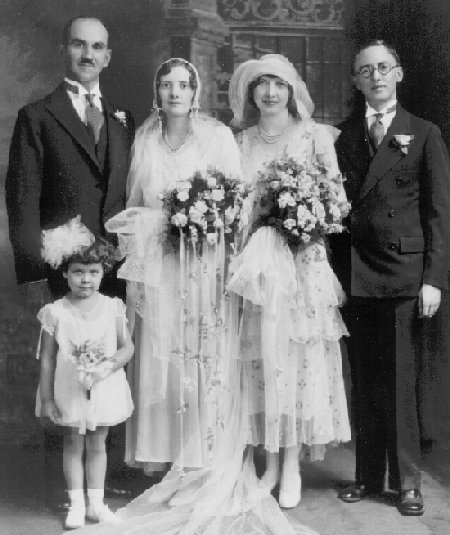## WeddingParty

Place mouse over face for identification of personDescription WeddingParty Date / Time 1930-08-11 Country / State / County / City / Placename USA, MN, StPaul Persons (t-b,l-r) Noah Louis Goulet, Florence Agnes Helsper, Leone Strange, John Schuller, Nona Dulin
```lib card:
dc:
title 		=	WeddingParty
creator 	=	Jerome Goulet
subject 	=
description 	=	Original (scanned analog or digital), names to faces, stored to LibCard "format", within image exif:
publisher 	=	Jerome Goulet
contributor 	=
date 		=	1930-08-11
resourcetype 	=
iformat 	=
if_mime 	=	image/jpeg
if_resolution 	=	72x72
if_dimensions 	=
if_dim_x 	=	450
if_dim_y 	=	535
resourceID 	=
source 		=	photograph (analog or digital)
language 	=	eng
rights 		=	Copyright 2020, Jerome Goulet<jtgoulet@yahoo.com>, GNU General Public License
lc:
when:
dateUTC		=	1930-08-11
timeUTC		=	0000
tz		=	+0000

dateLocal	=	1930-08-11
timeLocal	=	0000
where:
admin0		=	USA
admin1		=	MN
admin2		=	x
admin3		=	StPaul
pName		=	x
lat		=	x
lon		=	x
who:
person:
name:
surName		=	Goulet
givenName	=	Noah Louis
aka		=	NLG
relation	=
pip:	(person in picture)
circleX		=	88
circleY		=	52
circleRadius	=	43
boxX		=	59
boxY		=	23
boxWidth	=	58
boxHeight	=	58
label		=
uri		=
person:
name:
surName		=	Helsper
givenName	=	Florence Agnes
aka		=	FAH
relation	=
pip:	(person in picture)
circleX		=	175
circleY		=	91
circleRadius	=	34
boxX		=	152
boxY		=	68
boxWidth	=	46
boxHeight	=	46
label		=
uri		=
person:
name:
surName		=	Strange
givenName	=	Leone
aka		=	LS
relation	=
pip:	(person in picture)
circleX		=	271
circleY		=	90
circleRadius	=	45
boxX		=	241
boxY		=	60
boxWidth	=	60
boxHeight	=	60
label		=
uri		=
person:
name:
surName		=	Schuller
givenName	=	John
aka		=	JS
relation	=
pip:	(person in picture)
circleX		=	375
circleY		=	74
circleRadius	=	44
boxX		=	346
boxY		=	45
boxWidth	=	59
boxHeight	=	59
label		=
uri		=
person:
name:
surName		=	Dulin
givenName	=	Nona
aka		=	ND
relation	=
pip:	(person in picture)
circleX		=	86
circleY		=	277
circleRadius	=	30
boxX		=	66
boxY		=	257
boxWidth	=	40
boxHeight	=	40
label		=
uri		=
what:
eventName	=	WeddingParty
```